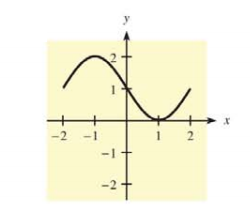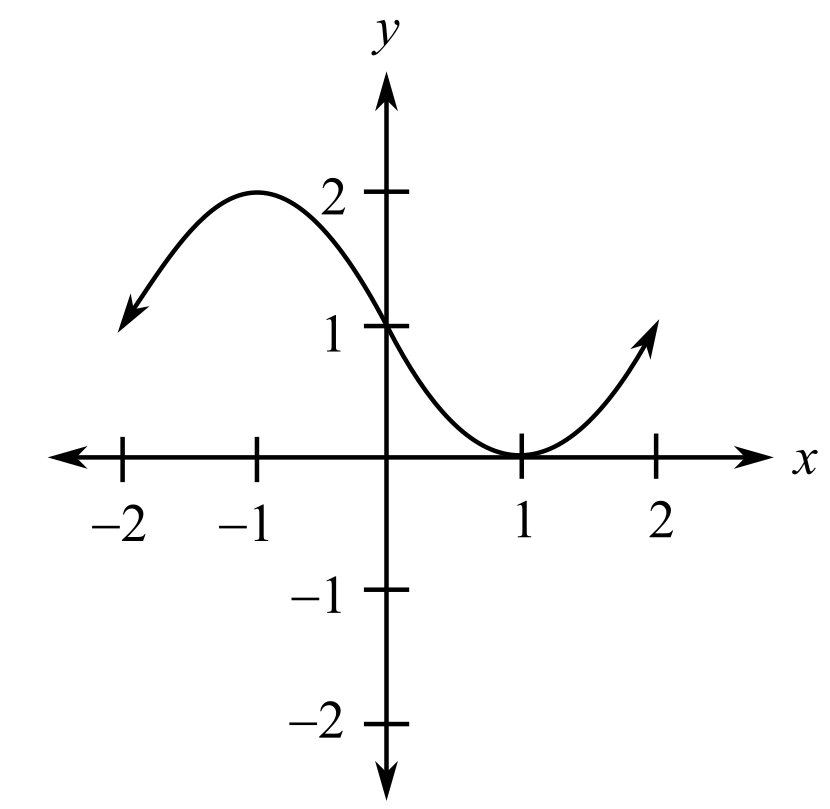Chapter 10.2, Problem 30E### Mathematical Applications for the ...

11th Edition
Ronald J. Harshbarger + 1 other
ISBN: 9781305108042

#### Solutions

Chapter
Section### Mathematical Applications for the ...

11th Edition
Ronald J. Harshbarger + 1 other
ISBN: 9781305108042
Textbook Problem

# In Problems 31 and 32, a graph is given. Tell where f(x) is concave up, where it is concave down, and where it has points of inflection on the interval — 2 < x < 2 if the given graph is the graph of ( a ) f ( x ) . ( b ) f ' ( x ) .     ( c ) f " ( x ) .(a)

To determine

To calculate: The region where the function f(x) is concave up, concave down and where the function has the points of inflection on the interval 2<x<2 if the given graph of function f(x).Explanation

Given Information:

The provided graph is

Formula used:

Concavity of f(x) can be found from the sign of f(x). Because f(x) is the first derivative of f(x), wherever the graph of f(x) is increasing, it follows that f(x)>0.

Thus, if f(x)>0 then f(x) is concave up

(b)

To determine

To calculate: The region where the function f(x) is concave up, concave down and where the function has the points of inflection on the interval 2<x<2 if the given graph of function f'(x).(c)

To determine

To calculate: The region where the function f(x) is concave up, concave down and where the function has the points of inflection on the interval 2<x<2 if the given graph of function f''(x).### Still sussing out bartleby?

Check out a sample textbook solution.

See a sample solution

#### The Solution to Your Study Problems

Bartleby provides explanations to thousands of textbook problems written by our experts, many with advanced degrees!

Get Started

#### Find more solutions based on key concepts